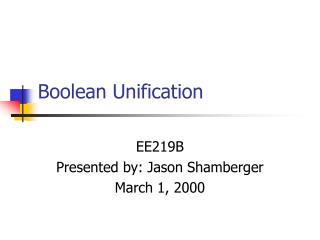DownloadDownload PresentationBoolean Unification

# Boolean Unification

Télécharger la présentation## Boolean Unification

- - - - - - - - - - - - - - - - - - - - - - - - - - - E N D - - - - - - - - - - - - - - - - - - - - - - - - - - -
##### Presentation Transcript

1. Boolean Unification EE219B Presented by: Jason Shamberger March 1, 2000

2. Outline • What is Boolean unification? • Boolean unification algorithms • Boole’s method • Löwenheim’s method • Applications

3. Boolean Unification • Boolean unification is a procedure to obtain a general solution of a Boolean equation • Use parametric variables to represent don’t cares

4. Boolean Unification • Given a Boolean equation of the form • Where xi’s are inputs and qi’s are constants • We want to find the most general substitution for xi’s, that satisfies the above equation

5. Boolean Unification • Where pi’s can be any variable or function

6. Boolean Unification: Example • Consider the following example: • The solutions for x1, x2, x3 are:

7. Algorithms for Boolean Unification • There are two main algorithms for Boolean unification • Boole’s method • Löwenheim’s method • Both algorithms can be implemented using BDD operations

8. Boole’s Method • Based on successive elimination of variables • Define conjunctive eliminant (ECON) as:

9. Boole’s Method • If X, G, and P represent the vectors of inputs, parameters, and parametric functions, then the parametric general solution of the equation: • Is a system: • Such that the following conditions are satisfied:

10. Theorem • It can be shown that for a Boolean function f, for which a solution to f(x)=0 exists: • Where:

11. Proof: • A sketch of the proof: • To show S=P:

12. Proof: • Show for all a in B, there is a p in B: • Use the property that: • Simplifies to: • Which is satisfied for all p=a

13. Boole’s Method • Using this result, we can generate parametric equations for each of the functions gi • Consider a 3 variable function: • We first calculate the eliminants:

14. Boole’s Method • We can now generate functions for each input x: • And the parametric general solution is:

15. Boole’s Method: Example • As an example, consider the RST flip-flop, which is defined by the equations: • y is the present state, Y is the next state, R,S,T are the reset, set, and toggle inputs. • The first equation describes how the next state is determined, and the second equation is the constraint that only one input may be a 1 at any time

16. Boole’s Method: Example • We first need to solve the system for R,S, and T which we consider variables, in terms of y and Y which we regard as elements of B. • Where f is defined by:

17. Boole’s Method: Example • The eliminants are:

18. Boole’s Method: Example • We can now derive the parametric expressions for the general solution: • Any values can be chosen for pi’s. • For example, let p1=1, p2=y :

19. Boole’s Method: • The method of successive elimination demonstrates that we need at most n parameters, but possibly less as in the previous example. • Most unification algorithms are implementations of Boole’s method

20. Löwenheim’s Method: • Allows us to form a parametric general solution from any particular solution • Useful when it is trivial to find a particular solution, and constructing a general solution is inconvenient

21. Löwenheim’s Method: • Theorem: Let U be a particular solution of a consistent Boolean equation f(x)=0, and let P be an n-tuple of arbitrary parameters. Then a parametric general solution is given by: • Provided that:

22. Löwenheim’s Method: • Proof: • Using the previous definition, the following conditions must be satisfied:

23. Löwenheim’s Method: Example • Consider the RST flip-flop example from before. An easily obtained particular solution of the RST equation is:

24. Löwenheim’s Method: Example • There are three inputs, thus there are three parameters, we will call them p,q,r. • Using the previous theorem, the general solution is given by:

25. Löwenheim’s Method: Example • After some calculations we arrive at: • Notice that we generate n new parameters for an n variable equation.

26. Applications • Applications include: • Design rectification • Boolean matching • Minimizing Boolean relations

27. Application: Design Rectification • Problem: • A circuit has already been laid out, but small modifications are desired (design errors, change in specs) • Can only add extra circuitry before input pins, or after output pins

28. Application: Design Rectification • To guarantee that the rectification is correct, the following must hold: • This is equivalent to computing: • Which is in the necessary form for Boolean unification

29. Conclusion • Boolean unification allows us to solve a Boolean equation of the form f(X)=0 • Generate parametric equations for each of the inputs xi • Is feasible to implement using BDD representations

30. References • Boolean Reasoning: The Logic of Boolean Equations. Brown, Frank Markham • Application of Boolean Unification to Logic Synthesis. Poncino, Massimo • Application of Boolean Unification to Combinational Logic Synthesis. Fujita, Masahiro etal.